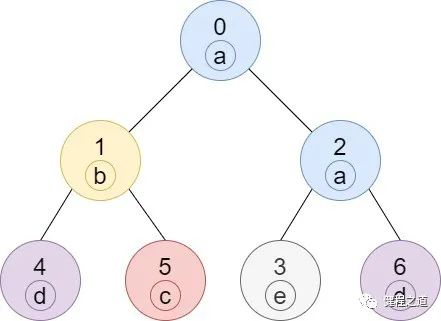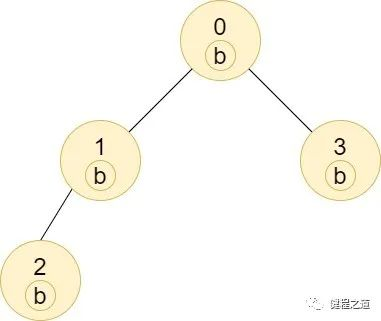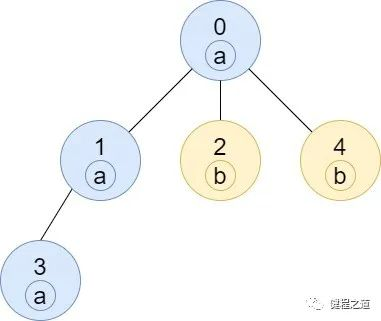# 力扣 1519——子树中标签相同的节点数

08/08 16:42

## 原题``输入：n = 7, edges = [[0,1],[0,2],[1,4],[1,5],[2,3],[2,6]], labels = "abaedcd"输出：[2,1,1,1,1,1,1]解释：节点 0 的标签为 'a' ，以 'a' 为根节点的子树中，节点 2 的标签也是 'a' ，因此答案为 2 。注意树中的每个节点都是这棵子树的一部分。节点 1 的标签为 'b' ，节点 1 的子树包含节点 1、4 和 5，但是节点 4、5 的标签与节点 1 不同，故而答案为 1（即，该节点本身）。````输入：n = 4, edges = [[0,1],[1,2],[0,3]], labels = "bbbb"输出：[4,2,1,1]解释：节点 2 的子树中只有节点 2 ，所以答案为 1 。节点 3 的子树中只有节点 3 ，所以答案为 1 。节点 1 的子树中包含节点 1 和 2 ，标签都是 'b' ，因此答案为 2 。节点 0 的子树中包含节点 0、1、2 和 3，标签都是 'b'，因此答案为 4 。````输入：n = 5, edges = [[0,1],[0,2],[1,3],[0,4]], labels = "aabab"输出：[3,2,1,1,1]``

``输入：n = 6, edges = [[0,1],[0,2],[1,3],[3,4],[4,5]], labels = "cbabaa"输出：[1,2,1,1,2,1]``

``输入：n = 7, edges = [[0,1],[1,2],[2,3],[3,4],[4,5],[5,6]], labels = "aaabaaa"输出：[6,5,4,1,3,2,1]``

• 1 <= n <= 10^5

• edges.length == n - 1

• edges[i].length == 2

• 0 <= ai, bi < n

• ai != bi

• labels.length == n

• labels 仅由小写英文字母组成

## 解题

### 首次尝试

``class Solution {    public int[] countSubTrees(int n, int[][] edges, String labels) {        // 构造树        LinkedList<Integer>[] tree = new LinkedList[n];        for (int[] edge : edges) {            // edge的子节点            LinkedList<Integer> child = tree[edge];            if (child == null) {                child = new LinkedList<>();                tree[edge] = child;            }            // 增加子节点            child.add(edge);        }        // 结果        int[] result = new int[n];        // 遍历并计算        for (int i = 0; i < n; i++) {            // 需要遍历的字符            char cur = labels.charAt(i);            // 该节点的子树中与该字符相同的节点数            int curCount = 0;            // 广度优先遍历            LinkedList<Integer> searchList = new LinkedList<>();            searchList.add(i);            while(!searchList.isEmpty()) {                int index = searchList.removeFirst();                if (cur == labels.charAt(index)) {                    curCount++;                }                // 找出该节点的子树                if (tree[index] == null) {                    continue;                }                searchList.addAll(tree[index]);            }            result[i] = curCount;        }        return result;    }}``

``输入：4[[0,2],[0,3],[1,2]]"aeed"输出：[1,2,1,1]预期：[1,1,2,1]``

``1   0 \ / \  2   3``

``    0   / \  2   3 /1``

### 双向记录构造树

``class Solution {    // 总节点数    int n;    // 树    Map<Integer, LinkedList<Integer>> tree;    // 字符串    String labels;    // 最终结果    int[] result;    public int[] countSubTrees(int n, int[][] edges, String labels) {        this.n = n;        this.labels = labels;        result = new int[n];        LinkedList<Integer> list;        // 双向构造树的关系        tree = new HashMap<>(n / 4 * 3 + 1);        for (int[] edge : edges) {            // 添加映射关系            list = tree.computeIfAbsent(edge, k -> new LinkedList<>());            list.add(edge);            list = tree.computeIfAbsent(edge, k -> new LinkedList<>());            list.add(edge);        }        // 深度优先搜索        dfs(0);        return result;    }    public int[] dfs(int index) {        // 当前子树中，所有字符的个数        int[] charArray = new int;        // 开始计算，标志该节点已经计算过        result[index] = 1;        // 获得其关联的节点        List<Integer> nodes = tree.get(index);        // 遍历        for (int node : nodes) {            // 如果该节点已经访问过            if (result[node] > 0) {                continue;            }            // 递归遍历子节点            int[] array = dfs(node);            for (int i = 0; i < 26; i++) {                charArray[i] += array[i];            }        }        // 将当前节点的值计算一下        charArray[labels.charAt(index) - 'a'] += 1;        result[index] = charArray[labels.charAt(index) - 'a'];        return charArray;    }}``

### 用空间换时间

``    public char charAt(int index) {        if ((index < 0) || (index >= value.length)) {            throw new StringIndexOutOfBoundsException(index);        }        return value[index];    }``

``class Solution {    // 总节点数    int n;    // 树    LinkedList<Integer>[] tree;    // 每个节点的值（用数字表示）    int[] nodeValueArray;    // 最终结果    int[] result;    public int[] countSubTrees(int n, int[][] edges, String labels) {        this.n = n;        nodeValueArray = new int[n];        result = new int[n];        // 双向构造树的关系        tree = new LinkedList[n];        for (int i = 0; i < n; i++) {            tree[i] = new LinkedList<>();        }        for (int[] edge : edges) {            // 添加映射关系            tree[edge].add(edge);            tree[edge].add(edge);        }        // 生成节点的值        for (int i = 0; i < n; i++) {            nodeValueArray[i] = labels.charAt(i) - 'a';        }        // 深度优先搜索        dfs(0);        return result;    }    public int[] dfs(int index) {        // 当前子树中，所有字符的个数        int[] charArray = new int;        // 开始计算，标志该节点已经计算过        result[index] = 1;        // 获得其关联的节点        List<Integer> nodes = tree[index];        // 遍历        for (int node : nodes) {            // 如果该节点已经访问过            if (result[node] > 0) {                continue;            }            // 递归遍历子节点            int[] array = dfs(node);            for (int i = 0; i < 26; i++) {                charArray[i] += array[i];            }        }        // 将当前节点的值计算一下        charArray[nodeValueArray[index]] += 1;        result[index] = charArray[nodeValueArray[index]];        return charArray;    }}``

### 研究一下目前最优解法

``public class Solution {  static class Next {    Next next;    Node node;    Next(Next next, Node node) {      this.next = next;      this.node = node;    }  }  static class Node {    /**     * 当前节点的index     */    final int index;    /**     * 当前节点对应的字符值（减去'a'）     */    final int ci;    /**     * 所有关联的节点     */    Next children;    /**     * 该节点的父节点     */    Node parent;    /**     * 子树中和该节点含有相同字符的节点总个数     */    int result;    /**     * 是否还在队列中，可以理解为是否已访问过     */    boolean inQueue;    public Node(int index, int ci) {      this.index = index;      this.ci = ci;      this.result = 1;    }    /**     * 从后往前，找到当前节点没有访问过的第一个子节点     */    Node popChild() {      for (; ; ) {        // 当前节点的所有关联节点        Next n = this.children;        // 如果没有，说明子节点都遍历完了        if (n == null) {          return null;        }        // 从后往前移除关联节点        this.children = n.next;        // 返回第一个没有访问过的节点        if (!n.node.inQueue) {          return n.node;        }      }    }    /**     * 访问了该节点     */    Node enqueue(Node[] cnodes) {      // 该节点标记为访问过      this.inQueue = true;      // 记录该节点的父节点      this.parent = cnodes[ci];      // 那么现在该字符值对应的最高节点，就是当前节点。      // 这样如果之后也遇到相同字符的子节点，就可以为子节点赋值其父节点，也就是上面一行是有效的      cnodes[ci] = this;      return this;    }    /**     * 退出该节点     */    void dequeue(Node[] cnodes, int[] res) {      // 之后会访问该节点的兄弟节点，因此父节点需要重新设置      cnodes[ci] = this.parent;      // 设置当前节点的值      res[index] = this.result;      // 父节点也可以进行累加      if (this.parent != null) {        this.parent.result += this.result;      }    }    void link(Node x) {      // this节点和x节点，互相绑定      this.children = new Next(this.children, x);      x.children = new Next(x.children, this);    }  }  public int[] countSubTrees(int n, int[][] edges, String labels) {    // 构造树    Node[] nodes = new Node[n];    // 每个节点对应的字符    for (int i = 0; i < n; i++) {      nodes[i] = new Node(i, labels.charAt(i) - 'a');    }    // 通过边的关系，将节点互相绑定    for (int[] es : edges) {      nodes[es].link(nodes[es]);    }    // 最终的结果    int[] res = new int[n];    // 当前访问的节点下标    int sz = 0;    // 26个小写英文字母对应的节点数组    Node[] cnodes = new Node;    // 下面三行可以合并成这一行：    // Node node = nodes[sz++] = nodes.enqueue(cnodes);    nodes[sz] = nodes.enqueue(cnodes);    // 当前访问的节点    Node node = nodes[sz];    // 因为当前节点已经访问过，自然下标需要+1    sz++;    for (; ; ) {      // 从后往前，找到当前节点没有访问过的第一个子节点      Node child = node.popChild();      // 如果已经全部访问过了      if (child == null) {        // 开始计算        node.dequeue(cnodes, res);        if (--sz == 0) {          break;        }        // 回溯到父节点        node = nodes[sz - 1];      } else {        // 保证了相邻节点一定是父子节点        node = nodes[sz++] = child.enqueue(cnodes);      }    }    return res;  }}``

## 总结

https://death00.github.io/0
0 收藏

### 作者的其它热门文章0 评论
0 收藏
0